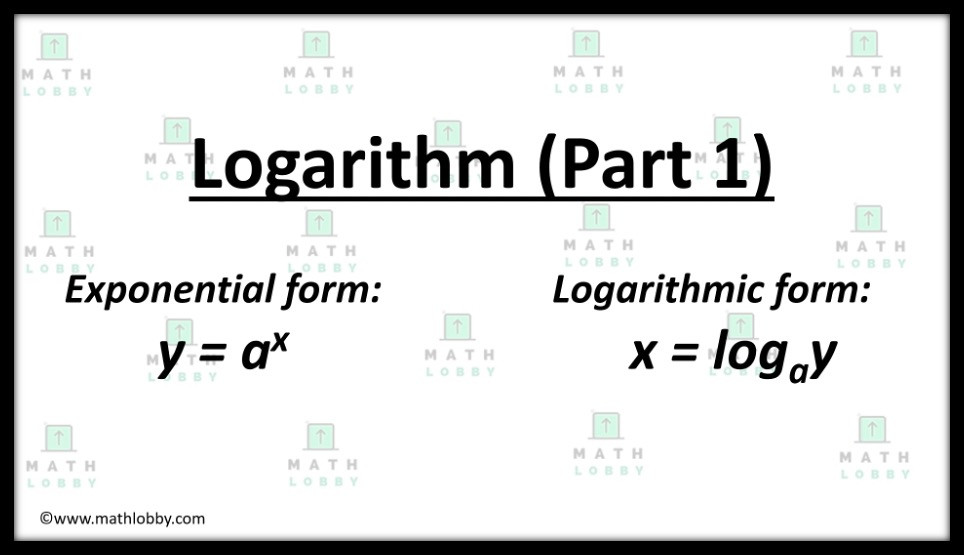Search

# Logarithm Part 1

Updated: Jun 22, 2021Dear students of Math Lobby, we will be covering Logarithm Part 1 today. Logarithm is covered in Additional Math (A Math) for Secondary 3 students.

Logarithm is a complex chapter to many students due to its unique laws. Hence, do pay close attention to what we are covering on today!

In this note, you will learn:

1) What is logarithm and how is it related to exponentials?

2) Relationship between laws of logarithm and indices

### 1) What is logarithm and how is it related to exponentials?

In the context of mathematics, logarithm is the inverse function to exponentiation. This means that the logarithm of a number or what we call index, x, is the exponent (power) of another fixed number, the base a, must be raised to in order to produce the number x.

Let’s take a look at how it works below:Note: The reason why a must always be a positive number (except for 1) is because if we take a to be 1, in its exponential form we will end up with 1 to the power of x, which will be 1 in any case.

This does not make any mathematical sense because we are will never be able to obtain the value of y regardless of the power we raise a to.

a can also never be 0, because similar to the reason given above, 0 to the power of x will still give us 0, which does not make any mathematical sense either.

a can also never be negative, because if we were to set the value of y to be ½, it will essentially be the square root of a negative number. In this context where complex numbers are not involved, this will also be deemed as mathematically illogical.

### 2) Relationship between laws of logarithm and indices

Since we now know the relationship between logarithm and exponential, there are certain laws in indices which can be adopted to suit logarithm. We can take a look at it below:

a) Power Law: (a^m)^n= a^(mn) -> loga x^r = r loga x

b) Product Law: a^m x a^n = a^(m+n) -> loga x + loga y = loga xy

c) Quotient Law: a^m/a^n = a^(m-n) -> loga x – loga y = loga (x/y)

d) Change of Base Law: loga b = (logc b)/(logc a)

Explanation:

a) Power Law

The first law we will be going through is the power law. Let’s take a look on how to proof it:

First of all, we will let loga x be equals to m,

So, in exponential form, it will be: a^m = x

Then, we raise the equation to the power of n, and then log both sides with the base a:

(a^m)n = xn

loga (a^(mn)) = loga (x^n)

Now, we will apply a special property of logarithm: When the argument of a logarithm is the same as the base, the answer will be 1. i.e. loga a = 1

Therefore, mn = loga x^n

And after we substitute loga x = m back into the equation, we will get:

n loga x = loga x^n

Example:

a) log4 64 = log4 (4^3)

= 3 log4 (4)

= 3

b) 4 + log3 (5)

= 4 log3 (3) + log3 (5)

= log3 (3^4) + log3 (5)

= log3 (81) + log3 (5)

= log3 (81 x 5)

= log3 (405)

b) Product Law

Next, we will be taking a look at the product law. Let’s see how its done:

Let loga x be equals to m, and loga y be equals to n,

In exponential form, it will look like this: a^m = x, a^n = y

Hence, xy = a^m x a^n

By law of indices: xy = a^(m+n)

And now we will log both sides of the equation with base a: loga (xy) = loga (a^(m+n))